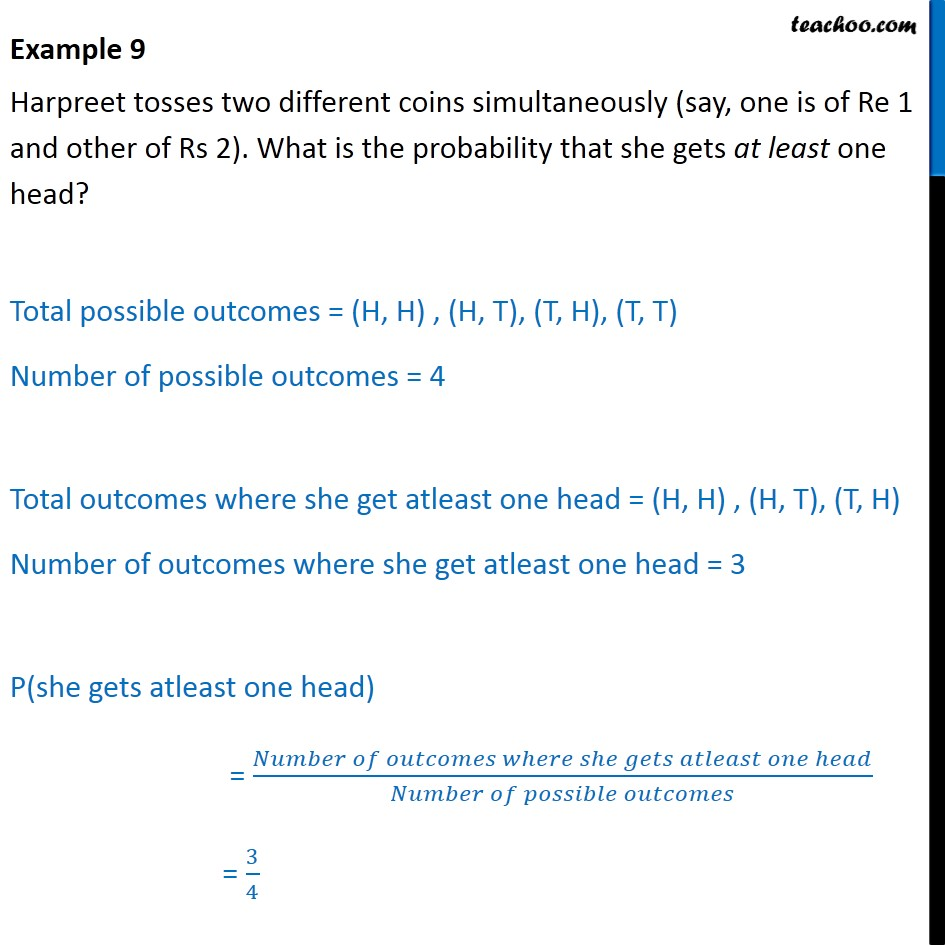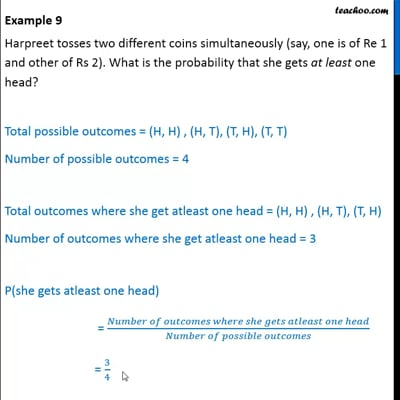Examples

Chapter 15 Class 10 Probability
Serial order wiseThis video is only available for Teachoo black users

Get live Maths 1-on-1 Classs - Class 6 to 12

### Transcript

Example 9 Harpreet tosses two different coins simultaneously (say, one is of Re 1 and other of Rs 2). What is the probability that she gets at least one head? Total possible outcomes = (H, H) , (H, T), (T, H), (T, T) Number of possible outcomes = 4 Total outcomes where she get atleast one head = (H, H) , (H, T), (T, H) Number of outcomes where she get atleast one head = 3 P(she gets atleast one head) = (𝑁𝑢𝑚𝑏𝑒𝑟 𝑜𝑓 𝑜𝑢𝑡𝑐𝑜𝑚𝑒𝑠 𝑤ℎ𝑒𝑟𝑒 𝑠ℎ𝑒 𝑔𝑒𝑡𝑠 𝑎𝑡𝑙𝑒𝑎𝑠𝑡 𝑜𝑛𝑒 ℎ𝑒𝑎𝑑)/(𝑁𝑢𝑚𝑏𝑒𝑟 𝑜𝑓 𝑝𝑜𝑠𝑠𝑖𝑏𝑙𝑒 𝑜𝑢𝑡𝑐𝑜𝑚𝑒𝑠) = 3/4